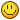# draw empty circle

## Recommended Posts

Hi I'd like to draw an empty circle like this one:I'm currently using the example from https://wiki.multitheftauto.com/wiki/DxDrawLine but it's not a perfect circle and yet very demanding.

I was wondering if there's a better way of doing this

Thanks• Moderators
3 hours ago, Tekken said:

very demanding

This one is less demanding:

3 hours ago, Tekken said:

I was wondering if there's a better way of doing this

Photoshop:

Create a png image (256 × 256), with.

• Giveblack borders.
• Fillwith a white colour if always filled, else use behind the image.

If filled with a white colour, it can be re-coloured with:

tocolor(255,255,255,255)

Create texture with mipmaps enabled:

What does mipmaps do?

Try to give the image

Edited by IIYAMA

dxDrawCircle returns a filled circle, the idea behind this is to create those circles as health indicators.

Haven’t tried making alpha 0 to center color tho

• Moderators
21 minutes ago, Tekken said:

dxDrawCircle returns a filled circle

Oh sorry, I meant this one:

but you still need that image to make the borders smooth.

Edited by IIYAMA
•1

Thanks!•1

try this

```function dxDrawCircle2( posX, posY, radius, width, angleAmount, startAngle, stopAngle, color, postGUI )
if ( type( posX ) ~= "number" ) or ( type( posY ) ~= "number" ) then
return false
end

width = type( width ) == "number" and width or 5
startAngle =  type( startAngle ) == "number" and startAngle or 0, 0, 360
stopAngle =  type( stopAngle ) == "number" and stopAngle or 360, 0, 360
color = color or tocolor( 255, 255, 255, 100 )
postGUI = type( postGUI ) == "boolean" and postGUI or false

startAngle = startAngle-90;
stopAngle = stopAngle-90;

if ( stopAngle < startAngle ) then
local tempAngle = stopAngle
stopAngle = startAngle
startAngle = tempAngle
end

local multiby = 50;

if (radius >= 1000) then multiby = 3000; end

for i = startAngle, stopAngle, multiby/radius do
local startX = math.cos( math.rad( i ) ) * ( radius - width )
local startY = math.sin( math.rad( i ) ) * ( radius - width )
local endX = math.cos( math.rad( i ) ) * ( radius + width )
local endY = math.sin( math.rad( i ) ) * ( radius + width )

dxDrawLine( startX + posX, startY + posY, endX + posX, endY + posY, color, width, postGUI )
end

return true
end```

i use this method with a circle image png

Edited by Furzy

## Create an account

Register a new account